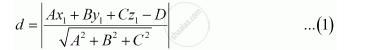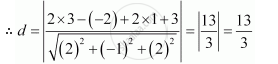Share

# In the Given Cases, Find the Distance of Each of the Given Points from the Corresponding Given Plane 2x – Y + 2z + 3 = 0 - CBSE (Science) Class 12 - Mathematics

ConceptDistance of a Point from a Plane

#### Question

In the given cases, find the distance of each of the given points from the corresponding given plane

Point                   Plane

(3, – 2, 1)             2x – y + 2z + 3 = 0

#### Solution

It is known that the distance between a point, p(x1y1z1), and a plane, Ax + By + Cz = D, is given by,The given point is (3, − 2, 1) and the plane is 2x – y + 2z + 3 = 0Is there an error in this question or solution?

#### APPEARS IN

NCERT Solution for Mathematics Textbook for Class 12 (2018 to Current)
Chapter 11: Three Dimensional Geometry
Q: 14.2 | Page no. 494

#### Video TutorialsVIEW ALL 

Solution In the Given Cases, Find the Distance of Each of the Given Points from the Corresponding Given Plane 2x – Y + 2z + 3 = 0 Concept: Distance of a Point from a Plane.
S